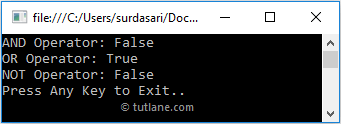# C# Logical Operators with Examples

In c#, Logical Operators are useful to perform the logical operation between two operands like AND, OR, and NOT based on our requirements. The Logical Operators will always work with Boolean expressions (true or false) and return Boolean values.

The operands in logical operators must always contain only Boolean values. Otherwise, Logical Operators will throw an error.

The following table lists the different types of operators available in c# logical operators.

OperatorNameDescriptionExample (a = true, b = false)
&& Logical AND It returns true if both operands are non-zero. a && b (false)
|| Logical OR It returns true if any one operand becomes a non-zero. a || b (true)
! Logical NOT It will return the reverse of a logical state that means if both operands are non-zero, it will return false. !(a && b) (true)

If we use Logical AND, OR operators in c# applications, those will return the result as shown below for different inputs.

Operand1Operand2ANDOR
true true true true
true false false true
false true false true
false false false false

If you observe the above table, if any one operand value becomes false, then the logical AND operator will return false. The logical OR operator will return true if any one operand value becomes true.

If we use the Logical NOT operator in our c# applications, it will return the results like as shown below for different inputs.

OperandNOT
true false
false true

If you observe the above table, the Logical NOT operator will always return the reverse value of the operand. If the operand value is true, then the Logical NOT operator will return false and vice versa.

## C# Logical Operators Example

Following is the example of using the Logical Operators in c# programming language.

using System;

namespace Tutlane
{
class Program
{
static void Main(string[] args)
{
int x = 15, y = 10;
bool a = true, result;
// AND operator
result = (x <= y) && (x > 10);
Console.WriteLine("AND Operator: " + result);
// OR operator
result = (x >= y) || (x < 5);
Console.WriteLine("OR Operator: " + result);
//NOT operator
result = !a;
Console.WriteLine("NOT Operator: " + result);
Console.WriteLine("Press Enter Key to Exit..");
}
}
}

If you observe the above code, we used logical operators (AND, OR, NOT) to perform different operations on defined operands.

## Output of C# Logical Operators Example

When we execute the above c# program, we will get the result as shown below.This is how we can use logical operators in the c# programming language to perform logical operations on defined operands based on our requirements.